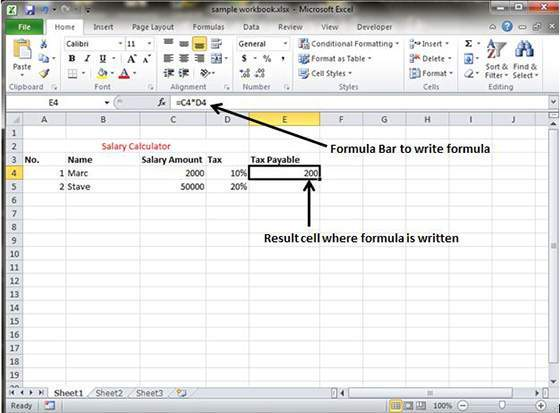# How to Create Formulas in MS Excel 2010

In order to create a formula in MS Excel 2010, you are simply required to the type in the formula bar. It should be noted that formula always begins with ‘=’ sign. While writing formulas manually, it can be typed either in the cell address or it can be pointed to them in the worksheet.

Most of the users find Pointing method to supply the cell addresses for formula lot easier and efficient in the worksheet. You can even make use of the built-in functions by simply clicking the cell or dragging through the cell range where formula is to be used. This snapshot will give a better idea about inserting formulas in the worksheet.Once you hit the enter key after inserting the formula, Excel will calculate the result and it will be displayed inside the cell. In case there is any error in the formula then Excel will able not be able to calculate the result at all. Instead an Alert dialog box will be displayed on the screen suggesting smart ways to fix the problem.